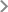CSU QR CodeEventsContent

Topic：The Orlicz-Minkowski Problem for Polytopes

Lecturer: Jiang Meiyue, School of Mathematical Science， Peking University

Time: May 4th 2017, 10:00-11:00 AM

Add:  Lecture Hall, Math Building, New Campus

Research Area:

Nonlinear, Hamilton system and Critical group theory

Synopsis:

The Orlicz-Minkowski problem for polytopes can be stated as follows: Given a function ?: (0,∞)→(0,∞). Let μ is a finite Borel measure on S^(n-1), which is not supported on a closed hemi-sphere, find a convex body K such that c ?(h_K)dS_K=dμ for some constant c>0, that is, for any Borel ω?S^(n-1), there holds c∫_ω?(h_K)dS_K=μ(ω). In this talk we will present some results for μ=∑a_iδ(u_i). The results are based on a joint work with Chu Wang.

close